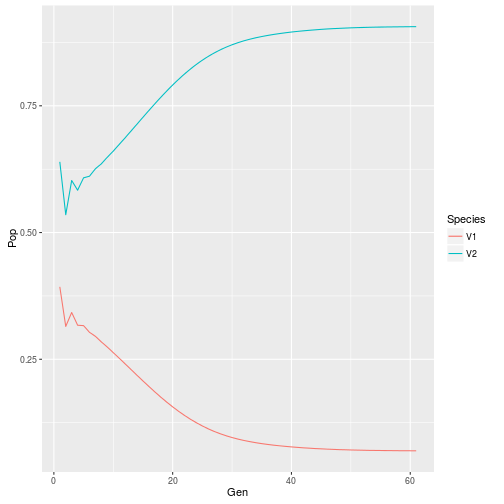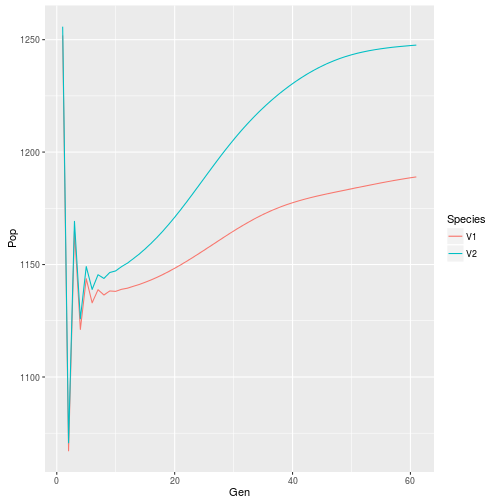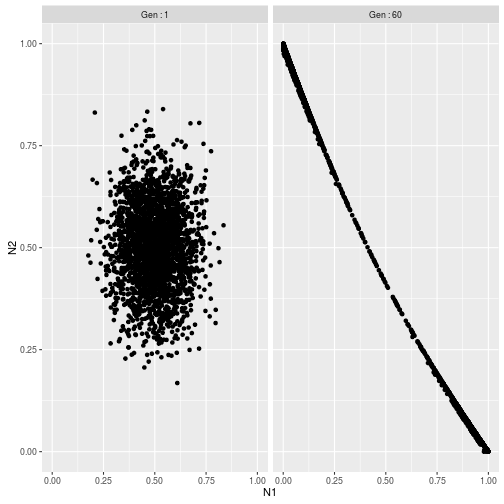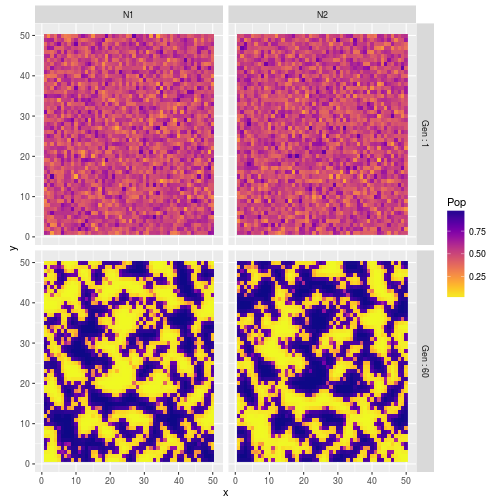Exploring Spatial Patterns and Coexistance

Implementation of the Coupled Map Lattice model presented in “Self-Organization in Complex Ecosystems” Solé & Bascompte (2006) Chp 3, page 87.

library(plyr)
library(reshape2)
library(ggplot2)
library(viridis)

#Lotka-Voltera discrete time competition function
#@Ns are the population abundance
#@rs are the growth rate
#@as is the competition matrix
discrete<-function(Ns,rs,as){
#return population abundance at time t+1
return(as.numeric(Ns*exp(rs*(1-as%*%Ns))))
}

#an helper function returning line numbers of all neighbouring cells
find_neighbour<-function(vec,dat=dat){
lnb<-which(dat[,"x"]%in%(vec["x"]+c(-1,-1,-1,0,1,1,1,0)) & dat[,"y"]%in%(vec["y"]+c(-1,0,1,1,1,0,-1,-1)))
#remove own line
lnb<-lnb[which(lnb!=vec["lnb"])]
return(lnb)
}

Look at the dynamic for two species with equal growth rate, equal dispersion rate and equal interspecific competition.

set.seed(20160611)
generation<-1:60
dat<-expand.grid(x=1:50,y=1:50)
dat\$N1<-0.5+rnorm(2500,0,0.1)
dat\$N2<-0.5+rnorm(2500,0,0.1)
dat\$lnb<-1:2500
list_dat<-list(dat)
#competition parameters
as<-matrix(c(1,1.2,1.2,1),ncol=2,byrow=TRUE)
#growth rate
rs<-c(1.5,1.5)
#dispersal rate
ds<-c(0.05,0.05)
#infos on neighbouring cells
neigh<-apply(dat,1,function(x) find_neighbour(x,dat))
#model the dynamic assuming absorbing boundary (ie ind that go out of the grid are lost to the system)
for(i in generation){
list_dat[[(i+1)]]<-rbind.fill(apply(list_dat[[i]],1,function(x){
#population dynamics within one grid cell
intern<-(1-ds)*discrete(Ns=x[c("N1","N2")],rs=rs,as=as)
#grab the neighbouring cells
neigh_cell<-list_dat[[i]][neigh[[x["lnb"]]],]
#the number of immigrant coming into the focal grid cell
imm<-(ds/8)*rowSums(apply(neigh_cell,1,function(y) discrete(Ns=y[c("N1","N2")],rs=c(1.5,1.5),as=as)))
out<-data.frame(x=x["x"],y=x["y"],N1=intern+imm,N2=intern+imm,lnb=x["lnb"])
return(out)
}))
#print(i)
}

#look at coexistence between the two species within one cell
cell525<-ldply(list_dat,function(x) c(x[525,"N1"],x[525,"N2"]))
cell525\$Gen<-1:61
cell525<-melt(cell525,id.vars="Gen",measure.vars=1:2,variable.name="Species",value.name="Pop")
ggplot(cell525,aes(x=Gen,y=Pop,color=Species))+geom_path()#look at coexistence across the system
all<-ldply(list_dat,function(x) c(sum(x[,"N1"]),sum(x[,"N2"])))
all\$Gen<-1:61
all<-melt(all,id.vars="Gen",measure.vars=1:2,variable.name="Species",value.name="Pop")
ggplot(all,aes(x=Gen,y=Pop,color=Species))+geom_path()#compare species-species abundance correlation at the beginning and at the end of the simulation
exmpl<-rbind(list_dat[],list_dat[])
exmpl\$Gen<-rep(c("Gen : 1","Gen : 60"),each=2500)
ggplot(exmpl,aes(x=N1,y=N2))+geom_point()+facet_grid(.~Gen)#look at variation in spatial patterns at the beginning and the end of the simulation
exmplm<-melt(exmpl,id.vars=c("x","y","Gen"),measure.vars=c("N1","N2"),variable.name="Species",value.name="Pop")
ggplot(exmplm,aes(x,y,fill=Pop))+geom_raster()+scale_fill_viridis(option="plasma",direction=-1)+facet_grid(Gen~Species)